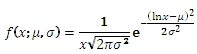# SQL Server LOGNORM.DIST function

LOGNORMPDF

Updated: 31 July 2010

Use LOGNORMPDF to calculate the probability density function of the lognormal distribution.

The formula for the probability density function is:Syntax
SELECT [wctStatistics].[wct].[LOGNORMPDF] (
<@X, float,>
,<@Mean, float,>
,<@Standard_dev, float,>)
Arguments
@X
is the variable to be evaluated. @X is an expression of type float or of a type that implicitly converts to float
@Mean
is the mean of the variable’s natural logarithm. @Mean is an expression of type float or of a type that implicitly converts to float
@Standard_dev
is the standard deviation of the variable’s natural logarithm. @B is an expression of type float or of a type that implicitly converts to float
Return Types
float
Remarks
·         @X must be greater than zero (@X > 0).
·         @Standard_dev must be greater than zero (@Standard_dev > 0).
·         Use LOGNORMDIST to calculate the upper cumulative distribution.
Examples
Calculate the probability density function:
SELECT wct.LOGNORMPDF(1,0,1)
This produces the following result
----------------------
0.398942280401433

(1 row(s) affected)

You can use the SeriesFloat function from the XLeratorDB/math library to generate a dataset which can be pasted into EXCEL to generate a graph of the probability density function.
SELECT SeriesValue
,wct.LOGNORMPDF(SeriesValue, 0, 2) as [f(x,0,2)]
,wct.LOGNORMPDF(SeriesValue, 0, 1) as [f(x,0,1)]
,wct.LOGNORMPDF(SeriesValue, 0, .5) as [f(x,0,.5)]
,wct.LOGNORMPDF(SeriesValue, 0, 0.25) as [f(x,0,0.25)]
,wct.LOGNORMPDF(SeriesValue, 0, 0.125) as [f(x,0,.125)]
FROM wctMath.wct.SeriesFloat(0.01, 3, .01,NULL,NULL)
This is an EXCEL-generated graph of the results### SupportCopyright 2008-2021 Westclintech LLC         Privacy Policy        Terms of Service